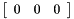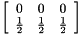FOX/ObjCryst++  1.10.X (development)
ObjCryst::SpaceGroup Class Reference

The crystallographic space group, and the cell choice. More...Collaboration diagram for ObjCryst::SpaceGroup:

## Classes

struct  SMx
Struct to store rot+trans matrix. More...

struct  TRx
Struct to store trans matrix. More...

## Public Member Functions

SpaceGroup ()
Default Constructor (initializes in P1) More...

SpaceGroup (const string &spgId)
Constructor with a specified spacegroup symbol or number. More...

~SpaceGroup ()
Destructor.

void ChangeSpaceGroup (const string &spgId)
Change the Spacegroup.

const string & GetName () const
Get the name of this spacegroup (its name, as supplied initially by the calling program or user)

bool IsInAsymmetricUnit (const REAL x, const REAL y, const REAL z) const
Test if a given scatterer at (x,y,z) is in the asymmetric unit.

void ChangeToAsymmetricUnit (REAL x, REAL y, REAL z) const
Move (x,y,z) coordinates to their equivalent in the asym unit. More...

const AsymmetricUnitGetAsymUnit () const
Get the AsymmetricUnit for this spacegroup.

int GetSpaceGroupNumber () const
Id number of the spacegroup.

bool IsCentrosymmetric () const
Is the crystal centrosymmetric ?

int GetNbTranslationVectors () const
Number of translation vectors (1 for 'P' cells, 2 for 'I', 4 for 'F',etc..) More...

const std::vector
< SpaceGroup::TRx > &
GetTranslationVectors () const
Return all Translation Vectors, as a 3 columns-array. More...

const std::vector
< SpaceGroup::SMx > &
GetSymmetryOperations () const
Get all symmetry operations stored in vector of struct SMx.

CrystMatrix_REAL GetAllSymmetrics (const REAL x, const REAL y, const REAL z, const bool noCenter=false, const bool noTransl=false, const bool noIdentical=false) const
Get all equivalent positions of a (xyz) position. More...

void GetSymmetric (unsigned int i, REAL &x, REAL &y, REAL &z, const bool noCenter=false, const bool noTransl=false, const bool derivative=false) const
Get all equivalent positions of a (xyz) position. More...

int GetNbSymmetrics (const bool noCenter=false, const bool noTransl=false) const
Return the number of equivalent positions in the spacegroup, ie the multilicity of the general position. More...

void Print () const
Prints a description of the spacegroup (symbol, properties). More...

bool HasInversionCenter () const
Is centrosymmetric ?

bool IsInversionCenterAtOrigin () const
Is the center of symmetry at the origin ?

const cctbx::sgtbx::space_group & GetCCTbxSpg () const
Get the underlying cctbx Spacegroup object.

const RefinableObjClockGetClockSpaceGroup () const
Get the SpaceGroup Clock (corresponding to the time of the initialization of the SpaceGroup)

unsigned int GetUniqueAxis () const
Which is the unique axis (for monoclinic space groups )

char GetExtension () const
Extension to space group symbol ('1','2':origin choice ; 'R','H'=rhomboedral/hexagonal)

CrystVector_REAL GetInversionCenter () const
Get the inversion center.

unsigned int AreReflEquiv (const REAL h1, const REAL k1, const REAL l1, const REAL h2, const REAL k2, const REAL l2) const
Are these reflections equivalent ? More...

CrystMatrix_REAL GetAllEquivRefl (const REAL h, const REAL k, const REAL l, const bool excludeFriedelMate=false, const bool forceFriedelLaw=false, const REAL sf_re=0, const REAL sf_im=0) const
Get the list of all equivalent reflections. More...

bool IsReflSystematicAbsent (const REAL h, const REAL k, const REAL l) const
Is the reflection systematically absent ?

bool IsReflCentric (const REAL h, const REAL k, const REAL l) const
Is the reflection centric ?

unsigned int GetExpectedIntensityFactor (const REAL h, const REAL k, const REAL l) const
Get the "expected intensity factor" for a given reflection. More...

## Private Member Functions

void InitSpaceGroup (const string &spgId)
Init the spaceGroup object from its name. More...

## Private Attributes

string mId
Spacegroup's name ( 'I422', 'D2^8','230') Maybe we should only store the Hermann-Mauguin symbol, rather than storing the string which was initially given by the user/program for the initialization. More...

cctbx::sgtbx::space_group * mpCCTbxSpaceGroup
SgOps structure for this spacegroup. More...

bool mHasInversionCenter
Is spacegroup centrosymmetric ? More...

bool mIsInversionCenterAtOrigin
Is center of symmetry at the origin ? More...

AsymmetricUnit mAsymmetricUnit
The spacegroup asymmetric unit.

RefinableObjClock mClock
The Spacegroup clock.

unsigned int mUniqueAxisId
Unique axis number (0=a,1=b,2=c)

unsigned long mNbSym
Number of symmetry operations (excluding center, and translations).

unsigned long mNbTrans
Number of lattice translations, including (0,0,0).

unsigned long mSpgNumber
SpaceGroup Number.

char mExtension
Extension to space group symbol (1,2:origin choice ; R,H=rhomboedral/hexagonal)

std::vector< SMxmvSym
Store floating-point matrices for faster use.

std::vector< TRxmvTrans
Store floating-point translation vectors for faster use.

## Detailed Description

The crystallographic space group, and the cell choice.

This class includes functions to get basic information about the symmetries, as well as getting all symmetrics for a given position in a unit cell.

This class included a pointer to a function calculating the "geometrical structure factor" (ie the sum of sin() and cos() for all symetrics, as could be found in the old version of the (red) International Tables), which was used to speed up computation of structure factors by using pre-factorised formulas. This is not used anymore, since methods can be used to speed up computations.

This class uses R. Grosse-Kunstleve 'SgLite' package, which is part of the Pymol package : http://pymol.sourceforge.net/

Warning
: the interface of the class will somewhat change when switching from sgLite to cctbx (http://cctbx.sourceforge.net). Particularly functions Spacegroup::GetSgOps() and Spacegroup::GetHM_as_Hall() will be removed.

Definition at line 104 of file SpaceGroup.h.

## Constructor & Destructor Documentation

 ObjCryst::SpaceGroup::SpaceGroup ( )

Default Constructor (initializes in P1)

You can use later SpaceGroup::ChangeSpaceGroup() to set the spacegroup.

Definition at line 212 of file SpaceGroup.cpp.

 ObjCryst::SpaceGroup::SpaceGroup ( const string & spgId )

Constructor with a specified spacegroup symbol or number.

Parameters
 spgId The space group identifier, either an Hermann-Maugin, or Hall, or Schonflies symbol.

Definition at line 217 of file SpaceGroup.cpp.

## Member Function Documentation

 unsigned int ObjCryst::SpaceGroup::AreReflEquiv ( const REAL h1, const REAL k1, const REAL l1, const REAL h2, const REAL k2, const REAL l2 ) const

Are these reflections equivalent ?

Returns
1 if they are equivalent, 2 if they are Friedel/Bijvoet mates, and else 0.

Definition at line 481 of file SpaceGroup.cpp.

 void ObjCryst::SpaceGroup::ChangeToAsymmetricUnit ( REAL x, REAL y, REAL z ) const

Move (x,y,z) coordinates to their equivalent in the asym unit.

Warning
Not implemented yet.
Todo:
SpaceGroup::IsInAsymmetricUnit()

Definition at line 240 of file SpaceGroup.cpp.

 CrystMatrix_REAL ObjCryst::SpaceGroup::GetAllEquivRefl ( const REAL h, const REAL k, const REAL l, const bool excludeFriedelMate = false, const bool forceFriedelLaw = false, const REAL sf_re = 0, const REAL sf_im = 0 ) const

Get the list of all equivalent reflections.

Returns
a matrix with 5 columns for h,k,l,Re(F),Im(F) and as many rows as there are reflections (the input reflection is included), with the associated structure factor, from the structure factor of the input reflection.
Parameters
 excludeFriedelMate if true, then Friedel mates of reflections will not be listed, even if there is a center of symmetry. forceFriedelLaw if true, a center of symmetry will be added (to force considering Friedel mates as equivalent). This as no effect if excludeFriedelMate=true sf_re,sf_im the real & imaginary part of the structure factor of the original reflection

Definition at line 514 of file SpaceGroup.cpp.

 CrystMatrix_REAL ObjCryst::SpaceGroup::GetAllSymmetrics ( const REAL x, const REAL y, const REAL z, const bool noCenter = false, const bool noTransl = false, const bool noIdentical = false ) const

Get all equivalent positions of a (xyz) position.

Parameters
 x,y,z fractional coordinates of the position noCenter if set to 'false' (the default), then the center of symmetry (if any) is used to generate ALL positions. If 'true', then only one half of equivalent positions are generated. This has no influence if the group is not centrosymmetric. (note Not generating symmetrical positions from center of symmetry is useful to speed up computation of structure factor, but is a bit tricky if the inversion is not at the origin. This is taken into account) noTransl if set to 'false' (the default), then translation are taken into account to generate all atom positions. This affect only body or face(s)-centered spacegroups. noIdentical if set to true, then atom in special positions will only return the distinct atomic positions. Currently two atoms are considered distinct if the difference for all of their fractionnal coordinates is less than 1e-5
Returns
a 3-column (x,y,z) matrix with as many rows as symmetric atoms
Warning
'special' positions are not taken into account. (ie an atom in special position will return duplicate entries. This may be corrected automatically later.) You can use the 'noIdentical' option for that,

Definition at line 274 of file SpaceGroup.cpp.

 unsigned int ObjCryst::SpaceGroup::GetExpectedIntensityFactor ( const REAL h, const REAL k, const REAL l ) const

Get the "expected intensity factor" for a given reflection.

This is the number of times the reflection is identical to the reflections deduced by the symmetry operators, under all distinct pure rotationnal symmetry operations of the space group.

This is used for the probability distribution of reflection intensities.

See:

• Stewart & Karle, Acta. Cryst 132 (1976), 1005
• Wilson, Acta Cryst 3 (1950), 258

Definition at line 562 of file SpaceGroup.cpp.

 int ObjCryst::SpaceGroup::GetNbSymmetrics ( const bool noCenter = false, const bool noTransl = false ) const

Return the number of equivalent positions in the spacegroup, ie the multilicity of the general position.

Parameters
 noCenter if 'true', do not take into account the center of symmetry noTransl if 'true', do not take into account translations

Definition at line 419 of file SpaceGroup.cpp.

 int ObjCryst::SpaceGroup::GetNbTranslationVectors ( ) const

Number of translation vectors (1 for 'P' cells, 2 for 'I', 4 for 'F',etc..)

Definition at line 259 of file SpaceGroup.cpp.

 void ObjCryst::SpaceGroup::GetSymmetric ( unsigned int i, REAL & x, REAL & y, REAL & z, const bool noCenter = false, const bool noTransl = false, const bool derivative = false ) const

Get all equivalent positions of a (xyz) position.

Parameters
 x,y,z fractional coordinates of the position. On return, these will contain the new values. noCenter if set to 'false' (the default), then the center of symmetry (if any) is used to generate ALL positions. If 'true', then only one half of equivalent positions are generated. This has no influence if the group is not centrosymmetric. (note Not generating symmetrical positions from center of symmetry is useful to speed up computation of structure factor, but is a bit tricky if the inversion is not at the origin. This is taken into account) noTransl if set to 'false' (the default), then translation are taken into account to generate all atom positions. This affect only body or face(s)-centered spacegroups. derivative if true, then this is used to calculate the displacement vector of s symmetric, given the orginal (dx,dy,dz)=> the translation components of gliding axis/plane are then ignored.

Definition at line 366 of file SpaceGroup.cpp.

 const std::vector< SpaceGroup::TRx > & ObjCryst::SpaceGroup::GetTranslationVectors ( ) const

Return all Translation Vectors, as a 3 columns-array.

The first vector is always [0,0,0]

Returnsfor a 'P' Cell,for a 'I' cell, andfor a 'F' cell,etc...

Definition at line 264 of file SpaceGroup.cpp.

 void ObjCryst::SpaceGroup::InitSpaceGroup ( const string & spgId )
private

Init the spaceGroup object from its name.

Initialize the SgOps & HM_as_Hall structures (SgLite), and set the function pointers to the functions used to compute the geometrical structure factors.

Definition at line 573 of file SpaceGroup.cpp.

 void ObjCryst::SpaceGroup::Print ( ) const

Prints a description of the spacegroup (symbol, properties).

Todo:

Definition at line 434 of file SpaceGroup.cpp.

## Member Data Documentation

 bool ObjCryst::SpaceGroup::mHasInversionCenter
private

Is spacegroup centrosymmetric ?

Definition at line 318 of file SpaceGroup.h.

 string ObjCryst::SpaceGroup::mId
private

Spacegroup's name ( 'I422', 'D2^8','230') Maybe we should only store the Hermann-Mauguin symbol, rather than storing the string which was initially given by the user/program for the initialization.

Definition at line 305 of file SpaceGroup.h.

 bool ObjCryst::SpaceGroup::mIsInversionCenterAtOrigin
private

Is center of symmetry at the origin ?

Definition at line 322 of file SpaceGroup.h.

 cctbx::sgtbx::space_group* ObjCryst::SpaceGroup::mpCCTbxSpaceGroup
private

SgOps structure for this spacegroup.

(Symmetry operations)

See sglite subdirectory for more information. This is (c) R. Gross-Kunstleve, part of PyMol software http://pymol.sourceforge.net/

Definition at line 313 of file SpaceGroup.h.

The documentation for this class was generated from the following files: